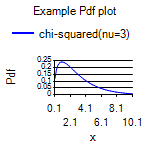/

### The Nematrian website: Expression Evaluation

One of the tools available on the Nematrian website is its Expression Evaluator. This tool allows users to use formulae rather than just numbers as inputs to online computations.

As with interactive use of individual Nematrian function webpages, the results are typically returned in the form of a hyperlink that embeds the input parameters used to carry out the calculation, to make it easier to check, reproduce or modify calculations at a later date. If results are charts then the same hyperlink embedding is also typically used. For example, if you wanted to move the legend in the following chart from above the figure to below it you could do so by clicking on the chart, altering the relevant ChartFormat parameter and then pressing the recalculate button on the page you landed on when you clicked on the chart.

There are four main ways of using the Expression Evaluator:

(a)    Insert an expression into the text box on the expression evaluator page and then press Calculate. This will then evaluate the expression within the text box.

(b)   Insert an expression into the Search / Calculate textbox at the top of any page on the website and press Calc button next to it. This replicates (a) but without needing to go to expression evaluator page beforehand.

(c)    Enter an expression into an input text box in the interactive input table on any page summarising a specific Nematrian web function having checked the corresponding box under the column headed “An expression input?” and then press the Calculate button. The relevant input element of the Nematrian function is then taken as the output of the relevant expression. The page relating to any particular Nematrian function can be found from the list of Nematrian web functions or by searching the Nematrian website).

(d)   Use an expression as an input to the (Mn)Evaluate function (and set its Language input parameter to blank) as this effectively replicates the output of the expression evaluator.

Formulae input into the expression evaluator are interpreted using a relatively simple syntax in line with typical algebraic conventions, i.e.:

-          Recognised algebraic operators are + (plus), - (minus), * (multiply), / (divide) and ^ (power).

-          Recognised logical operators are TRUE and FALSE, “>” (greater than), “<” (less than), “>=” (greater than or equal), “<=” (less than or equal), “=” (equals), “And” (logical and), “Or” (logical or), “XOr” (logical exclusive or, i.e. true if either x or y is true but not if both of them are true) and “Not”

-          Order of evaluation is (i) Brackets (i.e. “(“ and “)”) and function evaluation, see below), (ii) power, (iii) mutiply and divide, then (iv) add and subtract

-          All Nematrian Web functions are recognised, either including their leading “Mn” or without this prefix. Parameters follow immediately afterwards in brackets, with consecutive input parameters (if any) separated by commas. If an input parameter is an array then it should be enclosed in brackets with consecutive elements separated by commas.

-          Any dates and times should be entered either using the format #YYYY-MM-DD# or #YYYY-MM-DDTHH:MM:SS# or using Nematrian functions that return values that are Dates, e.g. (Mn)DateSerial,  (Mn)TimeSerial and (Mn)DateAndTimeSerial.

-          Integer values are automatically converted to Floating Point (i.e. “Double” aka Double Precision) values if necessary.

Note

(1)    Websites / browsers can be cautious if you enter “<” and “>” into text boxes (because it is possible to make malicious use of inputs that include these characters). If you come across this problem with this website then you can work around this issue by using the GT, GTE, LT and LTE functions, which return equivalent logical results to the >, >=, < and <= operators.

(2)    It is not currently possible to use the expression evaluator recursively within itself. Functions such as (Mn)Evaluate, (Mn)EvaluateUsing, (Mn)EvaluateMultiValues, (Mn)EvaluateExpressionsUsing and (Mn)EvaluateExpressionsMultiValues will not therefore be evaluated by the expression evaluator. Neither will plotting functions that make use of the expression evaluator such as (Mn)PlotExpression, (Mn)PlotExpressions and (Mn)PlotXYExpressionGeneral. Other more specialist plotting functions such as (Mn)ProbDistPlotPdf can be called via the expression evaluator, as illustrated below:Important notice

If you use any Nematrian web service either programmatically or interactively then you will be deemed to have agreed to the Nematrian website License Agreement.